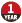# 数字图像处理学习——（一）双线性差值算法

2020-01-22 21:42:10  阅读：53  来源： 互联网

# 一、线性差值x1,x0,y1,y0为定值，可将上式变换为：
$y(x1-x0)=(x1-x)y0+(x-x0)y1$y(x1−x0)=(x1−x)y0+(x−x0)y1

# 二、双线性差值P的值可视作P1与P2的线性差值，而P1又可视作A,B的线性差值，P2可视作C,D的线性差值。
$P1(x1-x0)=A(x1-x)+B(x-x0)$P1(x1−x0)=A(x1−x)+B(x−x0);
$P2(x1-x0)=C(x1-x)+D(x-x0)$P2(x1−x0)=C(x1−x)+D(x−x0);

$b=(y-y0)/(y1-y0)$b=(y−y0)/(y1−y0);

$P1=A(1-a)+B*a$P1=A(1−a)+B∗a;
$P2=C(1-a)+D*a$P2=C(1−a)+D∗a;

P可视作P1与P2的线性差值

$P=A(1-a)(1-b)+B*a(1-b)+C(1-a)b+D*a*b$P=A(1−a)(1−b)+B∗a(1−b)+C(1−a)b+D∗a∗b杨少侠qy 发布了11 篇原创文章 · 获赞 18 · 访问量 8190